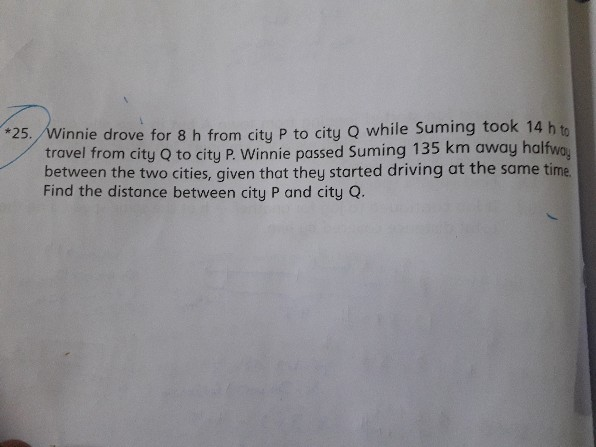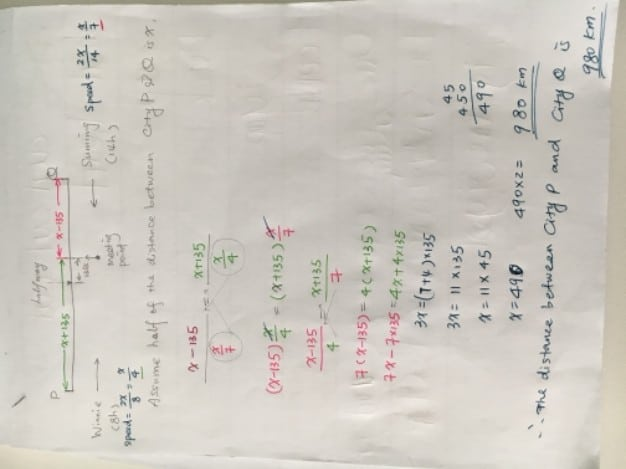QuestionPlease solve this problem

We can apply the following logic :

1 ) When the distance travelled by 2 objects is the same, the ratio in terms of time taken will be the opposite of the ratio in terms of speed

2) When the time taken is the same, the ratio in terms of speed will be the same as the ratio in terms of distance travelled

The first clue is “Winnie drove 8 hours from City P to City Q while Suming took 14 hours to travel from City Q to City P”

Since the distance travelled by Winnie and Suming is the same, we can form a ratio relationship between time taken and speed. The ratio in terms of time for Winnie and Suming will be 8 : 14, therefore the ratio in terms of speed for Winnie and Suming will be 14 : 8.

The second clue is “Winnie passed Suming 135 km away halfway between the 2 cities” Based on this clue, we can conclude that Winnie travelled 270 km more than Suming.

135 km x 2 = 270 km

The third clue is “They started driving at the same time”

Since the time taken is the same, we can form a ratio relationship between speed and distance travelled. As the ratio in terms of speed for Winnie and Suming is 14 : 8, we can conclude that the ratio in terms of distance travelled for Winnie and Suming is also 14 : 8

Based on this ratio, we can observe that the distance travelled by Winnie is 6 units more than Suming, we can further infer the following :

6 units = 270km

270km ÷ 6 = 45 km (1 unit)

The distance between both cities will be equal to the total distance travelled by Winnie and Suming

22 x 45 = 990 (22 units)

ANS: 990 km

0 Replies 0 Likes0 Replies 0 Likes